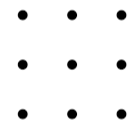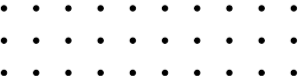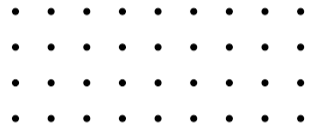#### You may also like### An Introduction to Magic Squares### Consecutive Negative Numbers

Do you notice anything about the solutions when you add and/or subtract consecutive negative numbers?### Always a Multiple?

Think of a two digit number, reverse the digits, and add the numbers together. Something special happens...

# Maxagon

##### Age 11 to 14Challenge Level

For this problem you may wish to download and print some dotty paper.
Alternatively, you could explore the problem using this interactivity.Draw some polygons by joining the dots on a $3$ by $3$ grid.

What is the greatest number of sides that your polygon could have?

What about on a $3$ by $4$ grid, or a $3$ by $5$ grid?

What about on a $3$ by $n$ grid?

Can you explain the pattern by which the 'number of sides' increases?Explore some polygons on grids that are $4$ dots high.

What is the maximum number of sides a polygon could have on a $4$ by $n$ grid?

Can you explain how you know?What is the maximum number of sides a polygon could have on a $6$ by $6$ grid?
And on a $6$ by $n$ grid?

With thanks to Don Steward, whose ideas formed the basis of this problem.